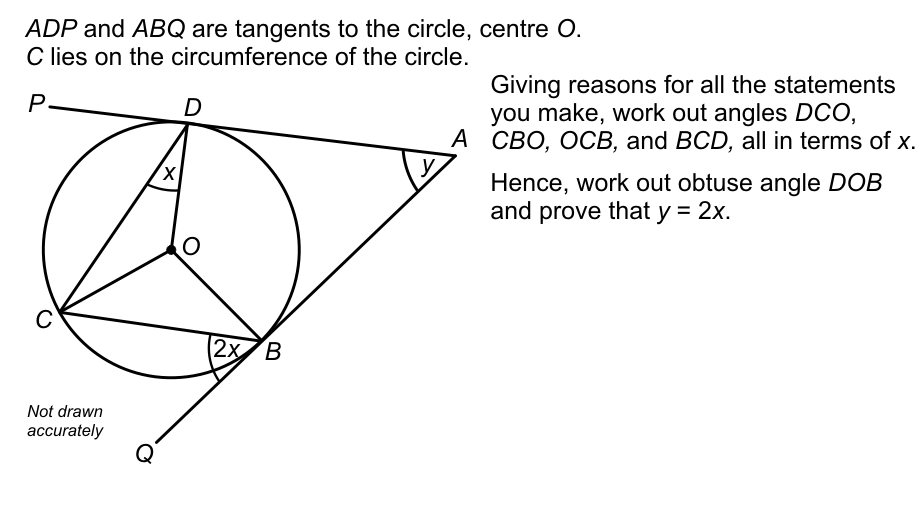Thread: I need help with circle theorums

1. I need help with circle theorums

Can i get help on this question. It is a 6 marker2. Re: I need help with circle theorums

Actually, I'd start backward a bit. Angles ADO and ABO are both right angles. (Prove this!) Hence you can get angle DOB in terms of y.

Can you fill in the steps?

-Dan

3. Re: I need help with circle theorums

it is really hard and I dont understand it

4. Re: I need help with circle theorums

Another hint then: Knowing that angle ABO is a right angle, then you know that 2x + angle CBO + 90 degrees = 180 degrees. (Again, prove it.) So what is angle CBO in terms of x?

Here's a site with a list of the circle theorems. Maybe having all of them in one spot might help.

-Dan

5. Re: I need help with circle theorumsOriginally Posted by Shookit is really hard and I dont understand it
Do you understand that $y=m(\angle BAD)=\frac{1}{2}\left( {arc(BCD) - arc(BD)} \right)~?$
The quadrilateral $ABOD$ contains two right angles & the sum of all its angles is $2\pi$. Therefore $m(\angle BOD)=\pi-y.$
Look at the figure: $m(\angle COD)=\pi-2x=m(arc DC)$; moreover since $2x=0.5(arc BC)$ then $4x=m(\angle COB)$.

$m(\angle BOC)+m(\angle COD)+m(\angle DOB)=2\pi$ now you finish and sow us your work.

Search Tags

cirle theorums, needhelpasap